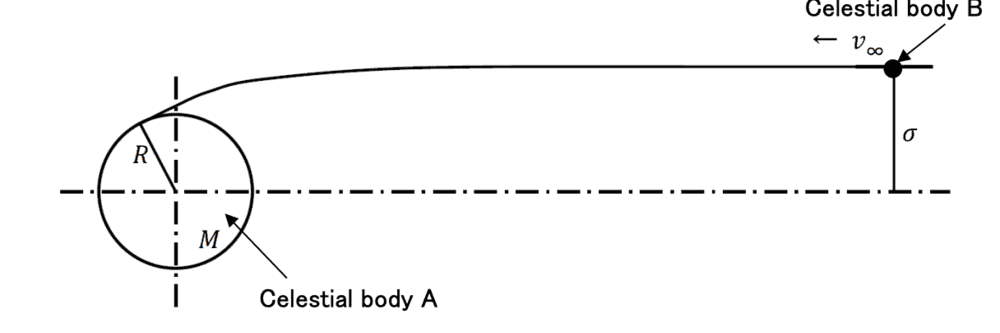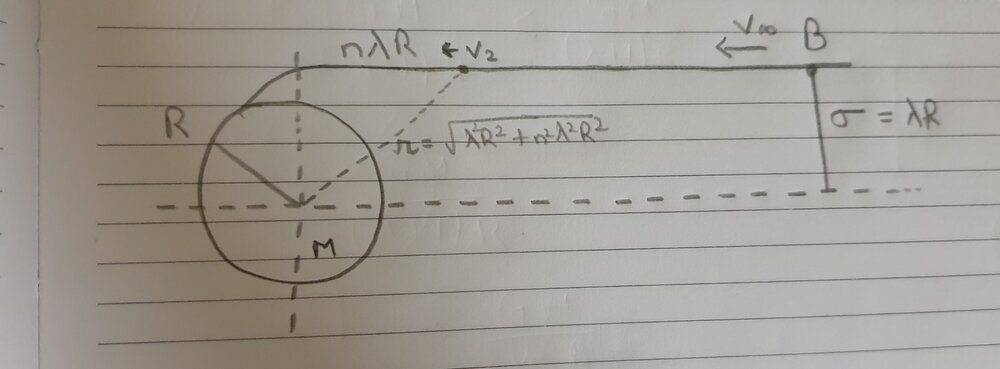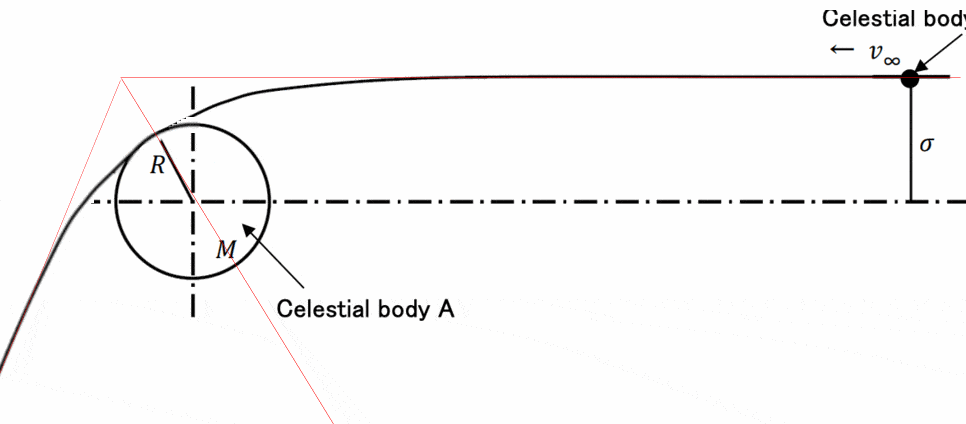# Gravitation: Object moving from infinity

• TopsyKrate
In summary, the experts are discussing the condition of 𝜎 where the celestial body B collides against the celestial body A. They are solving for the hyperbola where the periapsis is equal to R and using conservation of angular momentum and energy to find the solution. However, the assumption of the velocity ever being below orbital velocity is incorrect.f

#### TopsyKrate

Homework Statement
There is a spherical celestial body A with the radius of 𝑅 and the mass of 𝑀. As
shown in figure, consider movement of a celestial body B of which relative
velocity at infinity is 𝑣∞ with respect to the celestial body A and the mass is
negligible compared to the celestial body A. At infinity, the celestial body B moves
in the horizontal direction shown in the Figure, and the distance from the straight
line which passes through the center of the celestial body A and is parallel to the
movement direction of the celestial body B

Express the condition of 𝜎 where the celestial body B collides against the
celestial body A
Relevant Equations
GM/R
Express the condition of 𝜎 where the celestial body B collides against the
celestial body A

So this is the original figure of the problem.This is my attempt at a solutionSince I need to find σ, I have assumed sigma to be an multiple of the radius ## R ##. So, let ## \sigma = \lambda R ##, where ## \lambda ## is some constant term. I have also assumed that the object ## B ## comes into the gravitational influence of object ## A ## when its a horizontal distance of ## n \lambda R ## away (n is some other constant term)

So, using Pythagoras theorem, the distance of object B ## r = \sqrt{{(\lambda R)}^2 + {(n \lambda R)}^2} ##. Substituting ## \sigma ## back, this implies ## r= \sigma \sqrt{n^2 + 1} ##

So, my approach is when object is at a distance ## r= \sigma \sqrt{n^2 + 1} ##, its velocity is ## v_2 ##.This velocity should be less than the orbital velocity at distance ## r ## for it to collide with planet A.

This velocity can be calculated using conservation of energy.

At infinity, ## T_0 = \frac{1}{2}m{v_{\inf}}^2 ##.

At point ## v_2 ##, ## T_1 = \frac{1}{2}m{v_2}^2 - \frac{GMm}{r} ##

Solving this we get ## {v_2}^2 = {v_{inf}}^2 + \frac{2GM}{r} ##. Since ## v_2 < v_{orb} ##

$$\Rightarrow {v_{inf}}^2 + \frac{2GM}{r} < \frac{GM}{r}$$.

$$\Rightarrow {v_{\inf}}^2 < - \frac{GM}{r}$$

$$\Rightarrow r < - \frac{GM}{{v_{\inf}}^2}$$

Substituting everything, I get a value for ## \sigma ## which has the constant term ## n ##. However, I'm not supposed to have ## n ## in the answer. What am I doing wrong?

#### Attachments

Last edited:
I see no advantage in considering that B is not always in A's gravitational field.
What conservation laws apply?

•etotheipi
It sounds like you are trying to find what is sometimes called the "impact parameter".

The path the object will follow is a hyperbola with the focus at the center of object A. I've modified the diagram to illustrate.You are solving for the hyperbola where the periapsis ( Closest approach to the center of A) is equal to R.
Your assumption of the velocity ever being below orbital velocity is incorrect. At no point of it's trajectory will object B ever even drop below escape velocity.

•etotheipi
You are solving for the hyperbola where the periapsis ( Closest approach to the center of A) is equal to R.
The problem is much easier than that.

•etotheipi
It is an interesting exercise to do the derive the hyperbola, but my method (which I think is the same as @haruspex's method) just involves writing down two equations from two conservation laws and eliminating one variable.

Try using conservation of angular momentum as well as conservation of energy.